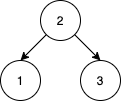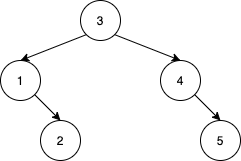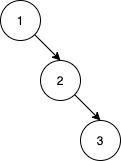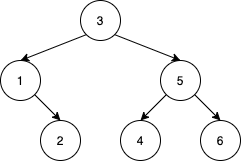# GeetCode Hub

Given an array `nums` that represents a permutation of integers from `1` to `n`. We are going to construct a binary search tree (BST) by inserting the elements of `nums` in order into an initially empty BST. Find the number of different ways to reorder `nums` so that the constructed BST is identical to that formed from the original array `nums`.

For example, given `nums = [2,1,3]`, we will have 2 as the root, 1 as a left child, and 3 as a right child. The array `[2,3,1]` also yields the same BST but `[3,2,1]` yields a different BST.

Return the number of ways to reorder `nums` such that the BST formed is identical to the original BST formed from `nums`.

Since the answer may be very large, return it modulo `10^9 + 7`.

Example 1:```Input: nums = [2,1,3]
Output: 1
Explanation: We can reorder nums to be [2,3,1] which will yield the same BST. There are no other ways to reorder nums which will yield the same BST.
```

Example 2:```Input: nums = [3,4,5,1,2]
Output: 5
Explanation: The following 5 arrays will yield the same BST:
[3,1,2,4,5]
[3,1,4,2,5]
[3,1,4,5,2]
[3,4,1,2,5]
[3,4,1,5,2]
```

Example 3:```Input: nums = [1,2,3]
Output: 0
Explanation: There are no other orderings of nums that will yield the same BST.
```

Example 4:```Input: nums = [3,1,2,5,4,6]
Output: 19
```

Example 5:

```Input: nums = [9,4,2,1,3,6,5,7,8,14,11,10,12,13,16,15,17,18]
Output: 216212978
Explanation: The number of ways to reorder nums to get the same BST is 3216212999. Taking this number modulo 10^9 + 7 gives 216212978.
```

Constraints:

• `1 <= nums.length <= 1000`
• `1 <= nums[i] <= nums.length`
• All integers in `nums` are distinct.

class Solution { public int numOfWays(int[] nums) { } }